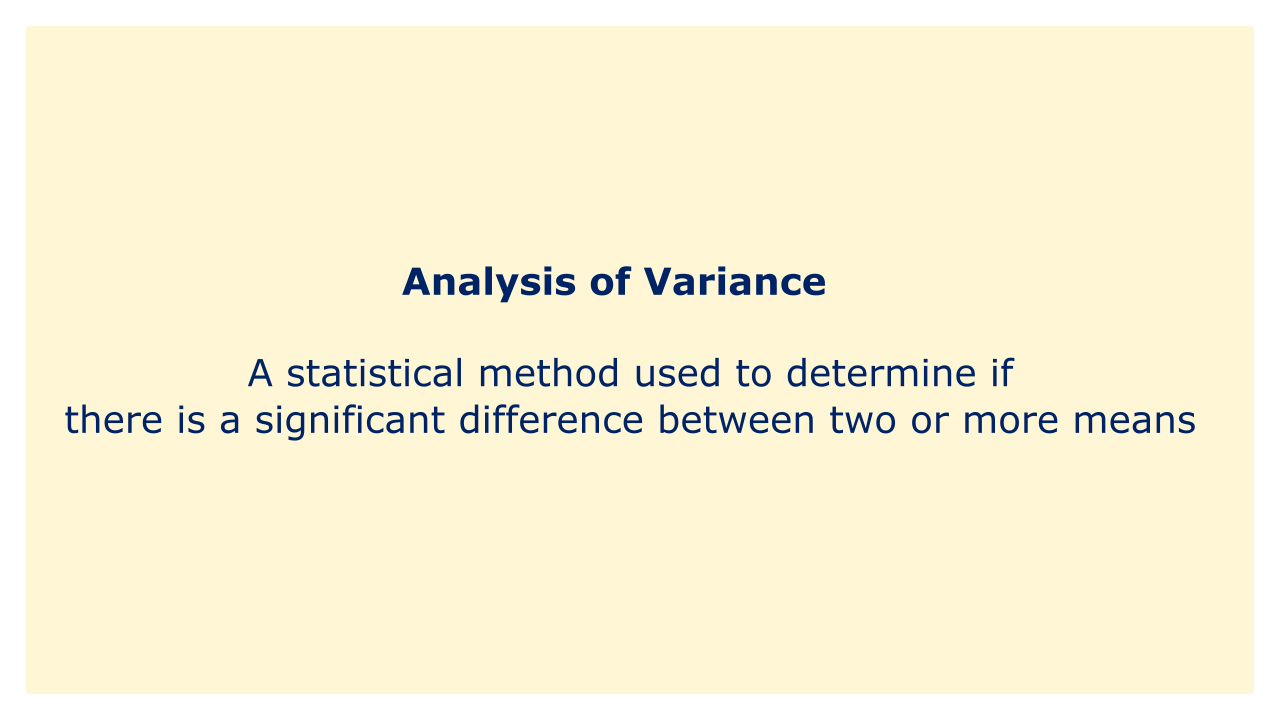# Analysis of VarianceImage: Moneybestpal.com

### A statistical technique called "analysis of variance" (ANOVA) is used to determine whether a difference between two or more means is significant. Testing hypotheses regarding the means of distinct populations or groups is a frequent practice in several academic disciplines, including psychology, sociology, biology, and economics.

The fundamental goal of an ANOVA is to contrast the variability within each group with that of the other groups. There may be a sizable discrepancy in the means of the groups if the variance within the groups is greater than the variance between the groups. If there is no discernible difference in the means of the groups, then the variability within the groups is greater than the variability between the groups.

There are several types of ANOVA, including one-way ANOVA, two-way ANOVA, and multi-way ANOVA. One-way ANOVA is used to test the difference between the means of two or more independent groups. Two-way ANOVA is used to test the difference between the means of two or more groups while considering the effect of two or more independent variables. Multi-way ANOVA is used to test the difference between the means of two or more groups while considering the effect of three or more independent variables.

In order to execute an ANOVA, the means of each group are calculated, and these means are then contrasted with the overall mean of the entire sample. The ratio of the variability between the groups to the variability within the groups is measured using a statistic known as the F-statistic, which is used in this comparison. If the F-statistic is high, it is likely that the group means differ significantly from one another.
Tags